## Linear Methods of Applied Mathematics Evans M. Harrell II and James V. Herod*

version of 28 January 2000

(Some remarks for the instructor).

You may wish to review the notion of a linear operator by referring to the Mathematica notebook for Chapter I.

## XIII. Geometry and integral operators

The following four problems illustrate, in a simple way, the primary concerns of the next several chapters. The first is a problem about matrices and vectors, and it will be our guide to solving integral equations and differential equations.

Then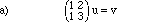if and only if u = B v.

The equivalence of these two matrix-vector equations is easy to establish, and you have no doubt learned long ago how to construct the inverse matrix B such that statement (b) is equivalent to statement (a).

Model Problem XIII.2. Find the inverse of an integral operator. Example: Let u and v be functions of x in the interval [0,1], and let K(x,t) := 1 + x t. The function u is a solution to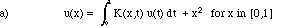if and only if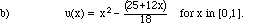If one supposes u is given by the formula (b), then the integral calculus will show that u satisfies (a). On the other hand, the task of deriving a formula for u from the relationship in (a) involves unfamiliar techniques, which we shall discuss in this course.

Model Problem XIII.3. Find the integral solution operator for an ordinary differential equation. Example: Let f and g be continuous functions of x in the interval [0,1], and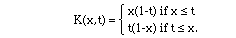The function g is a solution for

(a) g''= -f and g(0) = g(1) = 0

if and only if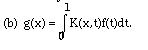Once again, the task of deriving this connection is probably somewhat mysterious, but the result can be checked with the calculus:

VERIFICATION OF MODEL PROBLEM 3.

(a)=>(b) Suppose that f is continuous on [0,1] and g'' = -f with g(0) = g(1) = 0. Suppose also that K is as given by sample problem (3). Then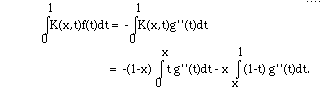Using integration by parts this last line can be rewritten as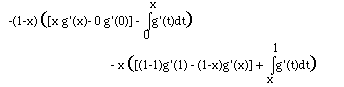= -(1-x)[x g'(x) -(g(x)-g(0)}
-x[-(1-x) g'(x) + (g(1) - g(x))]
= (1-x) g(x) + x g(x) = g(x).
To get the last line we used the assumption that g(1) = g(0) = 0.

(b)=>(a) Again, suppose that f is continuous and, now, suppose that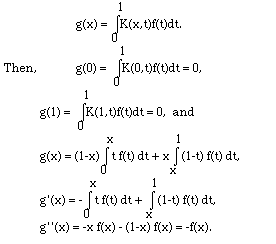As you can see, it is not hard to show that these two statements are equivalent. In the next few chapters you will learn how, given statement (a), you can construct K such that statement (b) is equivalent to statement (a). Perhaps you can do this already.

uxx + uyy = 0 for y > 0 and all x,
with boundary data that u(x,0) = sin(x), and the "condition at infinity" that u(x,y) remains finite as y ->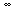.
The solution, as can be verified by elementary calculus, is u(x,y) = e-y sin(x). We shall later learn how to obtain such a solution by means of integral transforms of the boundary data.
One of the unifying ideas of this course is that each of these model problems can be written in the form Lu=v for a linear operator L. It is a worthwhile exercise to reformulate each of these model problems in this form.

Most often, we shall take the interval on which our functions are defined to be [0,1]. Of course, we do not work in the class of all functions on [0,1]; rather, in the spirit of Chapters I-II, we ask that the linear space should consist of functions f for which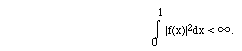Then we have the inner product space we called L2( [0,1] ) in Chapter II. We recall that the inner product of two functions is given by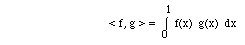and the norm of f is defined as the square-root of the inner product of a function with itself: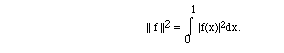(Compare with the norm in Rn.)

It does not seem appropriate to study in detail the nature of L2[0,1] at this time. Rather, suffice it to say that the space is large enough to contain all continuous functions - even functions which are continuous except at a finite number of places. The interested student can learn more about L2[0,1] by looking in standard books on real analysis or Hilbert space.

Suppose { fp } is a sequence of functions in L2( [0,1]). It is valuable to consider the possible meanings for the statement that limp fp(x) = g(x). There are three useful interpretations for our purposes:

• The sequence {fp} converges pointwise to g at each x in [0,1] provided that for each x in [0,1],        limp fp(x) = g(x).
Sometimes we modify this to convergence pointwise a.e. when the set on which the convergence fails is a null set.
• The sequence converges to g uniformly on [0,1] provided that

limp supx |fp(x) - g(x)| = 0.

• The sequence converges to g in norm if

limp || fp - g || = 0.

• Convergence in norm is the same as r.m.s. convergence, as described in chapter II. Uniform convergence on a finite interval implies both of the other two notions of convergence, but examples show that other implications among these notions are not generally valid. (Compare with the notions of convergence for sequences of vectors in Rn.)

In this section we study one of the most common types of integral equation. As an example, given a function called the kernel

K: [0,1]x[0,1] -> R

and a function f: [0,1] -> R, we seek a function y such that for each x in [0,1],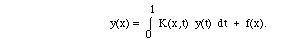Such equations are called Fredholm equations of the second kind. An equation of the form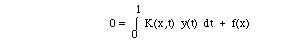is a Fredholm equation of the first kind.

The requirements in this section on K and f will be that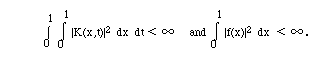These requirements are met if K and f are continuous.

For simplicity, we denote by K the linear function given by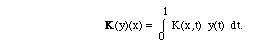Note that K has a domain large enough to contain all functions y which are continuous on [0,1]. Also, if y is continuous then K(y) is a function and its value at x is denoted K(y)(x). In spoken conversation, it is not so easy to distinguish the number valued function K and the function valued K. The bold character will be used in these notes to denoted the latter.

It is well to note the resemblance of this function K to the multiplication of a vector u by a matrix A: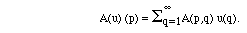This formula has the same form as that for K given above.

It is a historical accident that differential equations were understood before integral equations. Often an integral equation can be converted into a differential equation or vice versa, so many of the laws of nature which we think of as differential equations might just as well have been developed as integral equations initially. In some instances it is easier to differentiate than to integrate, but at other times integral operators are more tractable.

In this course integral operators will be called upon to solve differential equations, and this is one of their main uses. They have many other uses as well, most notably in the theory of filtering and signal processing. In most of these applications the integral and differential operators are linear transformations. The analogy between linear transformations and matrices is deep and useful.

In order to understand K*, one must consider < K(f), g > and seek K* such that < Kf, g > = < f, K*g >.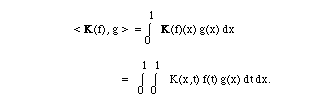An examination of these last equations leads one to guess that K* is given by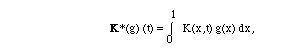or, keeping t as the variable of integration,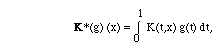Those last equations verified that

< K(f), g > = < f, K*(g) >.

Care has to be taken to watch whether the "variable of integration" is t or x in the integrals involved.

In summary, if K is the kernel associated with the linear operator K, then the kernel associated with K* is given by K*(x,y) [[equivalence]] K(y,x). It is of value to compare how to get K* from K with the process of how to get A* from A:

A*p,q = Aq,p.

Consistent with the rather standard notation we have adopted above, it is clear that a briefer representation of the equation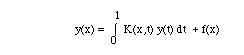is the concise equation y = K(y) + f, or (1 - K ) y = f.

Example XIII.5: Suppose that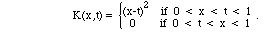To get K*, let's use other letters for the argument of K* and K to avoid confusion. Suppose that 0 < u < v < 1. Then, K*(u,v) = K(v,u) = 0. In a similar manner, K*(u,v) = (u-v)2 if 0 < v < u < 1. Note that K* is not K.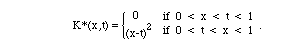The discussion of this example has been algebraic to this point. Consider this geometric notion that is suggested by the alternate name for "self-adjoint", namely, some call K "symmetric" if K(x,t) = K(t,x). The geometric name suggests a picture and the picture is the graph of K. The K of this example is not symmetric in x and t. Its graph is not symmetric about the line x = t. The function K is different from the function K*.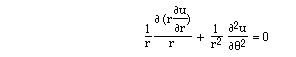THE FREDHOLM ALTERNATIVE THEOREMS

A first understanding of the problem of solving an integral equation

y = Ky + f

can be gotten by referring to the Fredholm Alternative Theorems in this context.

(Review the alternative theorem for matrices.)

I. Exactly one of the following holds:

(a)(First Alternative) if f is in L2{0,1}, then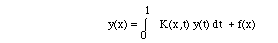has one and only one solution.

(b)(Second Alternative)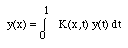has a nontrivial solution.

II. (a) If the first alternative holds for the equationthen it also holds for the equation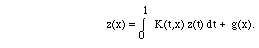(b) In either alternative, the equation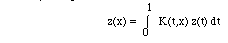have the same number of linearly independent solutions.

III. Suppose the second alternative holds. Then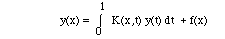has a solution if and only if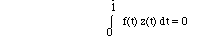for each solution z of the adjoint equation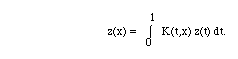Comparing this context for the Fredholm Alternative Theorems with an understanding of matrix examples seems irresistible. Since these ideas will re-occur in each section, the student should pause to make these comparisons.

Example XIII.6: Suppose that E is the linear space of continuous functions on the interval [-1,1]. with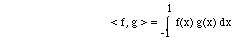and that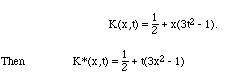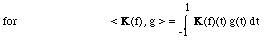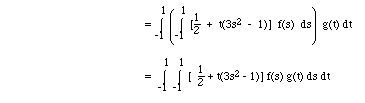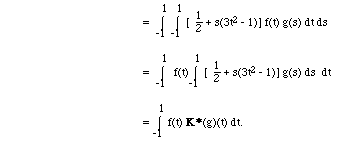The equation y = K(y) has a non-trivial solution: the constant function 1. To see this, one computes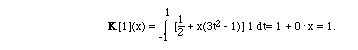One implication of these computations is that the problem y = Ky + f is a second alternative problem. It may be verified that y(x) = 1 is also a nontrivial solution for y = K*y. It follows from the third of the Fredholm alternative theorems that a necessary condition for y = Ky + f to have a solution is that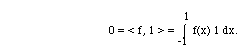Note that one such f is f(x) = x + x3.

Exercises.

XIII.1. Reformulate each of Model Problems XIII.2-XIII.4 in the form Lu = v. I.e., carefully identify the to which u and v belong, as well as how the operator L acts. For Model Problem XIII.4 make v a nonzero quantity related to the boundary condition sin(x) by choosing the vector spaces for the linear operator intelligently.

XIII.2.    Suppose K(x,t) =1 + 2 x t2 on [0,1]x[0,1] and y(x) = 3 - x. Compute    K(y) and K*(y). Ans: (5+3x)/2,                 (15+14x2)/6

XIII.3. Let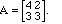Find B such that, if v is in R2, then these are equivalent:

(a) u is a vector and Au = v.

(b) v is a vector and u = Bv.

XIII.4. Let K be as in Model Problem XIII.2. Show that if u(x) = 3x2 - (25 + 12x )/6 then u solves the equation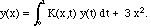XIII.5. Let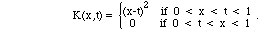Suppose that f is continuous on [0,1]. Show these are equivalent: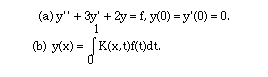XIII.6. Let u(r,= r sin(). Show thatwith u(1,) = sin().

XIII.7..   Suppose K(x,t) = x t if 0 < x < t < 1, and = x t2 if 0 < t < x < 1)). For y(x) = 3 - x, compute K(y)    and K*(y).

Ans: K[y](x) = - x5/4 + 4x4/3 - 3x3/2 + 7x/6.

XIII.8.

(1) Suppose that E is the linear space of continuous functions on [0,1] with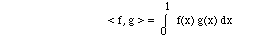and that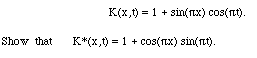(2) Show that y = Ky has non-trivial solution the constant function 1.

(3) Show that y = K*y has non-trivial solution the function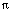+ 2 cos(x).

(4) What conditions must hold on f in order that

y = Ky + f

should have a solution?

Find examples of sequences of functions which converge

• pointwise but not uniformly
• pointwise but not in norm
• in norm but not pointwise
• in norm but not uniformly.